TitleBeginning Algebra
Tutorial 12: Addition Property of EqualityLearning Objectives

 After completing this tutorial, you should be able to: Know what a linear equation is. Know if a value is a solution or not. Use the addition and subtraction properties of equalities to solve linear equations.Introduction

 This is where we start getting into the heart of what algebra is about,  solving equations.  In this tutorial we will be looking specifically at linear equations and their solutions.  In this and the next tutorial, we will start off slow and solve equations that use only one property to make sure you have the individual concepts down.  Then, in later tutorials,  we will pick up the pace and mix 'em up where you need to use several properties and steps to get the job done. Equations can be used to help us solve a variety of problems.  The tutorial is ready when you are.Tutorial

 Equation Two expressions set equal to each other.

 Linear Equation  in One Variable An equation that can be written in the form  ax + b = c where a, b, and c are constants.

 Note that the exponent (definition found in Tutorial 4: Introduction to Variable Expressions and Equations) on the variable of a linear equation is always 1.

 The following is an example of a linear equation: 3x - 4 = 5

 Solution A value, such that, when you replace the variable with it, it makes the equation true. (the left side comes out equal to the right side)

 Solution Set Set of all solutions

 Solving a Linear Equation  in General Get the variable you are solving for alone on one side and everything else on the other side using INVERSE operations.

 Addition and Subtraction Properties of Equality If a = b, then a + c = b + c If a = b, then a - c = b - c

 In other words, if two expressions are equal to each other and you add or subtract the exact same thing to both sides, the two sides will remain equal. Note that addition and subtraction are inverse operations of each other.  For example, if you have a number that is being added that you need to move to the other side of the equation, then you would subtract it from both sides of that equation.Example 1:  Solve the equation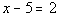.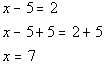*Inverse of sub. 5 is add. 5

 Note that if you put 7 back in for x in the original problem you will see that 7 is the solution to our problem.Example 2:  Solve the equation.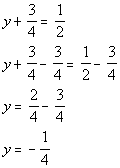*Inverse of add 3/4 is sub. 3/4  *LCD = 4 *1/2 = 2/4

 If you put -1/4 back in for y in the original problem you will see that -1/4 is the solution to our problem.Example 3:  Solve the equation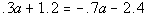.

 In this problem, our variable a is on both sides of the equation.  As mentioned above, when solving a linear equation you need to get the variable you are solving for alone on one side and everything else on the other side using INVERSE operations. At this point we are limited.  We only have talked about using the addition and subtraction properties of equality.  In Tutorial 13, we will address the multiplication and division properties of equality.  But since this was made before that, we have to make ado with addition and subtraction. We can solve this with what we know so far.  We move a term that has a variable exactly the same way we were moving constants in examples 1 and 2.  In this problem we need to get a on one side and everything else on the other.  We have a -.7a on the right side.  To move it to the other side, so a is only on one side, we will do the inverse of minus, which is add .7a to both sides.  After that it looks like examples 1 and 2 above, and we continue doing inverse operations until we have a on one side and everything on the other side of the equation. Let's see what we get: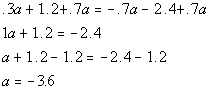*Inverse of sub.  .7a is add .7a *Inverse of add  1.2 is sub. 1.2

 If you put -3.6 back in for a in the original problem you will see that -3.6 is the solution to our problem.Example 4:  Solve the equation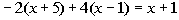In this problem, we need to simplify the left side first before we can start adding or subtracting things around.  We can accomplish this by using the distributive property (found in Tutorial 8: Properties of Real Numbers).   Using the distributive property and then combining like terms  to simplify the left side of the equation we get:*Use distributive prop. *Combine like terms

 Solving for x we get: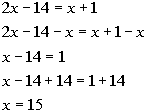*Inverse of add  x is sub. x *Inverse of sub. 14 is add. 14

 If you put 15 back in for x in the original problem you will see that 15 is the solution to our problem.Example 5:  Two numbers have a sum of 100.  If one number is x, express the other number in terms of x.

 Let’s put this one in terms that everyone can relate to, MONEY.  Let's say that you owe two people a total of \$100.  You owe the first person \$75.  How much do you owe the second person?  The answer would be \$100 - \$75 = \$25.  To figure it out you would take the total and then subtract out the known amount to get the other amount. We can use that concept to figure out our problem.  Anytime you know the total of two numbers,  you subtract the given from the total to either find the other number or express the other number in terms of a variable. Since our total is 100 and we are letting x represent one number, the other number would be expressed as the total minus x or 100 - x. So, 100 - x is our answer.Practice Problems

 These are practice problems to help bring you to the next level.  It will allow you to check and see if you have an understanding of these types of problems. Math works just like anything else, if you want to get good at it, then you need to practice it.  Even the best athletes and musicians had help along the way and lots of practice, practice, practice, to get good at their sport or instrument.  In fact there is no such thing as too much practice. To get the most out of these, you should work the problem out on your own and then check your answer by clicking on the link for the answer/discussion for that  problem.  At the link you will find the answer as well as any steps that went into finding that answer.Practice Problems 1a - 1d: Solve the given equation.

 1a.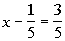(answer/discussion to 1a) 1b.(answer/discussion to 1b)

 1c.(answer/discussion to 1c) 1d.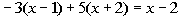(answer/discussion to 1d)Practice Problem 2a: Write an algebraic expression.

 2a.  Two numbers have a sum of 200.  If one number is x, express the other number in terms of x. (answer/discussion to 2a)Need Extra Help on these Topics?

 Go to Get Help Outside the Classroom found in Tutorial 1: How to Succeed in a Math Class for some more suggestions.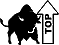Last revised on July 26, 2011 by Kim Seward.
All contents copyright (C) 2001 - 2010, WTAMU and Kim Seward. All rights reserved.